Courses

# CAT Quant Mock Test - 3

## 25 Questions MCQ Test Quantitative Aptitude (Quant) | CAT Quant Mock Test - 3

Description
This mock test of CAT Quant Mock Test - 3 for CAT helps you for every CAT entrance exam. This contains 25 Multiple Choice Questions for CAT CAT Quant Mock Test - 3 (mcq) to study with solutions a complete question bank. The solved questions answers in this CAT Quant Mock Test - 3 quiz give you a good mix of easy questions and tough questions. CAT students definitely take this CAT Quant Mock Test - 3 exercise for a better result in the exam. You can find other CAT Quant Mock Test - 3 extra questions, long questions & short questions for CAT on EduRev as well by searching above.
QUESTION: 1

### DIRECTIONS for questions: Select the correct alternative from the given choices. The function g(x) = |x – 4| + |4.5 – x| + |4.8 – x|, where x is a real number, attains its minimum value at (a)x = 4.1 (b)x = 4.4 (d)None of the above

Solution:

If 4 < x<4.5, g(x) = x- 4 + 4.5 -x + 4.8 - x = 5.3 - x

If 4.5

g(x) decreases with x for x < 4.5 and increases with x for 4.5 < x

∴g(x) has minimum value at x = 4.5

QUESTION: 2

### DIRECTIONS for questions: Select the correct alternative from the given choices. Each of the numbers a1, a2, a3, - - - an, where n ≥ 3m is equal to 1 or –1. Suppose a1 a2 a3 + a2 a3 a4 + a3 a4 a5 + - - - + an – 3 an – 2 an – 1 + an – 2 an – 1 an + an – 1 an a1 + an a1 a2 = 0, then

Solution: Each of the n terms of the sum a1, a2, a3 +a2 a3 a4 +- - - + an a1a2 is either 1 or-1 as each term is a product of 1’s and / or - 1’s.

Since the sum is zero the number of terms equal to 1 must be same as the number of terms equal to -1. This is possible only when ‘n’ is even.

Hence, n must be an even number.

QUESTION: 3

### DIRECTIONS for questions: Select the correct alternative from the given choices. There are five pipes P1, P2, P3, P4 and P5 which can fill a tank in 45, 30, 15, 10 and 9 minutes respectively. Exactly two of the pipes are now converted into emptying pipes retaining the same respective flow rates. If one filling pipe and one emptying pipe operate, the empty tank gets filled in 12 x 6 / 7 minutes, while for another combination of a filling pipe and an emptying pipe, the full tank gets emptied in 22 x 1 / 2 minutes. Which of the following pipes may have been converted into emptying pipes?

Solution:

Considering 90 as the capacity of the tank {LCM of (45, 30, 15, 10, 9) we can find therate of flow for the 5 pipes as 90 / 45,90 / 30, 90 / 15, 90 / 10 and 90 / 9 respectively. The time and the rates of the 5 pipes are tabulated below.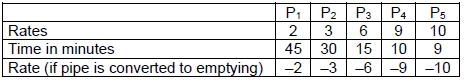If the tank gets filled in 12 x 6 / 7 min, effective rate of filing

= 90 / (90 / 7)= 7 units/min

If the tank gets emptied in 22 x 1 / 2min, effective rate of emptying = 90 / (45 / 2)= 4 units/min

7 units/min can be obtained by making P4 a filling pipe and P1 emptying pipe or Pe filling and P2 emptying. If P4 is filling pipe and P1 emptying pipe, 4 units/min can be obtained by making P5 emptying pipe and P3 filling pipe. In the latter case, P3 emptying and P1 filling pipe.

Case(i):7 = 10-3 = P5-P2

-4 = 2 - 6 = P1 - P3

Case(ii):7 = 9- 2 = P4 – P1

-4 = 6- 10 = P3-P5

∴P1, P5 or P3, P2 may have been converted to emptying pipes. Only P2, P3 is listed among the options.

QUESTION: 4

DIRECTIONS for questions: Select the correct alternative from the given choices.

If 2x + 3y > 6 and y – x > – 1, the sign of which of the following expressions can be uniquely determined?

I. 3x + 7y

II. 4x + 11y

III. x + y

Solution: We are given two inequalities of the same type, i.e., “greater than". We can multiply them by p and q respectively and add the resulting inequalities and get a new inequality, provided p and q have the same sign.

Further, the sign ofagiven expression oftheform ax + by can be determined for some cases of (a, b), where a, b are obtained by multiplying the first and second inequalities by p and q and adding the resulting inequalities (in the given question a = 2p - q and b- 3p + q) provided p, q have the same sign.

For the three given expressions, p, qr are tabulated below.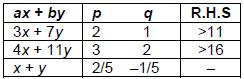We conclude that the sign of 3x + 7y and 4x + 11 y can be determined but not that of 5(x + y) or x + y.

QUESTION: 5

DIRECTIONS for questions: Select the correct alternative from the given choices.

A graph is defined as a set of points connected by lines called edges. Each edge connects a pair of points. It is possible to reach any point from any other point through a sequence of edges. Consider a graph with 15 points. If the number of edges in the graph is denoted by e, then which of the following best describese?

Solution: If a graph has N points, the number of edges has a minimum value of N - 1 and a maximum of N(N – 1) / 2

As N = 15, 14 ≤ e ≤ 105

QUESTION: 6

DIRECTIONS for questions: Select the correct alternative from the given choices.

If the equation (x – k)2 + (y + k)2 = 9, represents a circle, with centre O, in the co-ordinate plane and k is a positive constant, then which of the following is an appropriate representation of the graph in the co-ordinate plane?

Solution: (x - k2) + (y + k)2 = 9 is an equation of circle with centre at (k, - k) and radius 3 units. As k is positive, the centre of the circle lies in the 4thquadrant in which the x-coordinate is positive and the y-coordinate is negative.

QUESTION: 7

DIRECTIONS for question: Select the correct alternative from the given choices.

The median of a set of eight numbers is ‘m ’. If a number larger than the largest number in the set is included, the median increases by 4. If the largest number in the set is removed, the median becomes m / 2. Find the median, if a number smaller than the least number in the set is included.

Solution: Let T1, T2,……….T8 be the terms in ascending order.

Median = T4 + T5 / 2= m

=> T4 + T5 = 2m → (1)

When T9, a number greater than T8, is included, the median is T5.

∴T5 = m + 4→ (2)

when T8 is removed, the median is T4.

∴T4 = m / 3 → (3)

From (1), (2) and (3), we get

m + 4+ m / 2 = 2m=>4 = m / 2 => m = 8

When a number smaller than the least number is added, median = T4= m / 2 = 4

QUESTION: 8

DIRECTIONS for questions: Answer the questions on the basis of the information given below.

Ram Kumar wanted to come down from the first floor to the ground floor of a shopping mall, whereas Kishore wanted to climb up from the ground floor to the first floor. Both used the same escalator (a moving staircase) which was ascending from the ground floor to the first floor and both walked towards their respective destinations at their normal speeds. Both of them started simultaneously from the top and the bottom of the escalator respectively and crossed each other after exactly 21 seconds. If instead, Kishore had walked at 1/3rd of his normal speed while Ram Kumar maintained his normal speed, they would have crossed each other after exactly 28 seconds from the start. Further, if both Ram Kumar and Kishore had climbed up from the ground floor to the first floor using the same ascending escalator, walking at their normal speeds, the number of steps taken by Kishore to reach the first floor would be 20% less than the number of steps taken by Ram Kumar for the same.

Q.Ram Kumar walked down from the first floor to the ground floor using the same escalator. However, after some time the escalator stopped moving due to a power failure. Find the total time taken by Ram Kumar to reach the ground floor, given that the time for which he walked on the moving escalator was the same as that for which he walked on the stationary escalator.

Solution:

Let the speeds of Ram Kumar and Kishore be x steps per second and y steps per second respectively. Both having started simultaneously, the total length covered by them to cross each other must be equal to the total length of the escalator.

∴(x + y)21 = (x + y / 3) 28 = Number of steps on the escalator

∴ 9(x + y) = 4(3x + y)

5y = 3x

Y / x = 3 / 5

Let x = 5k and y = 3k,

Total steps in the escalator = 21 (5k + 3k) = 168k

Both having started from the ground floor in the time Ram kumar takes 5k1steps, Kishore will take 3k1 steps. So they will be separated by 2k1 steps.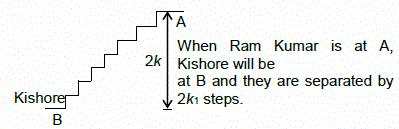Total steps taken by Kishore to reach the 1stfloor = 5K1 – 20 / 100 = 5k1 = 4k1

So in the time Kishore took kt steps, the escalator took the remaining (2k1– k1) steps.

Thus speed of Kishore = Speed of the escalator.

Speed of the escalator = 3k steps/seconds

Since for half the time Ram Kumar will be walking in opposite direction to that of the escalator, relative speed of Ram Kumar = 5k - 3k = 2k steps/seconds.

For the other half of the time, with the escalator being at rest Ram Kumar’s speed will be 5k steps/seconds.

Let Ram Kumar cover n steps at 2k steps/seconds speed and the remaining at 5k steps/seconds speed.

Since the time taken is same in both cases, n / 2k = 168k – n / 5k

5 n = 336k - 2n

7n = 336k or n = 48k

Time taken by Ram Kumar to reach the ground floor = 2 x n / 2k = 2 x 48k / 2k= 48 seconds

QUESTION: 9

DIRECTIONS for questions: Select the correct alternative from the given choices.

If set A contains five elements and set B contains four elements, how many different onto mappings from A to B are possible?

Solution: A function is said to be onto if each element in the range has at least one corresponding element in the domain.

=> Each of the four elements of B must have at least one corresponding element of A.

Two elements of A are mapped to one element of B. The remaining elements are mapped one each to the remaining elements of B.

Two elements from A can be selected in 5C2 ways and one element from B can be selected in 4C1 ways. The remaining three elements can be mapped in 3! ways.

Total ways = 5C2. 4C1 . 3! = 240

QUESTION: 10

DIRECTIONS for questions: Select the correct alternative from the given choices.

Spending Rs.422, Ranjit bought 35 pens from among three varieties of pens – A, B, C. If each pen of varieties A, B, and C costs Rs.10, Rs.8, and Rs.15 respectively, and Ranjit bought the maximum possible pens of variety C, find the total number of pens of varieties A and B that he bought.

Solution: Let the number of pens of type A, B and C bought by Ranjit be x, y and z respectively.

Given,

x + y + z = 35 → (1)

10x + 8y + 15z = 422 → (2)

8x + 8y + 8z = 280→ (3)

Subtracting (3) from (2), we get

2x + 7z = 142

The possible values of x and corresponding values of z have been substituted in the equation

x z y

142 = 2(15)+ 7(16) 4

= 2(8)+ 7(18)9

= 2(1)+ 7(20) 14

As he bought as many type C pens as possible (i.e. 20), he bought 15 pens of type A and B.

QUESTION: 11

DIRECTIONS for questions: Select the correct alternative from the given choices.

In a class of n students, the students who get more than 70% are awarded Distinction. Among these students, those who get more than 90% are also awarded a Certificate of Merit. In how many possible ways could Distinction and Certificates of Merit be secured by the students in the class?

Solution: For each student, there are three instances.

(i)less than 70%

(ii)more than 70% but not more than 90% (Distinction)

(iii)More than 90% (Distinction + certificate of Merit)

Hence each student can be selected in 3 ways.

For n students = 3x3x ……..n times = 3n

QUESTION: 12

DIRECTIONS for questions: Select the correct alternative from the given choices.

A parallelogram is divided into nine regions of equal area by drawing line segments parallel to one of its diagonals. What is the ratio of the length of the longest of the line segments to that of the shortest?

Solution: The lines must be drawn as follows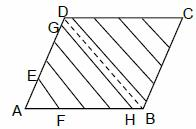As EF is parallel to GH, △AEF and △AGH are similar. As the area of each part is equal, let area of △AEF be x. Then area of △AGH = 4x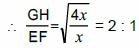QUESTION: 13

DIRECTIONS for questions: Select the correct alternative from the given choices.

A had a certain amount of money. B had seven times the amount that A had. A bought a certain number of gold coins and was left with one-third the cost of a gold coin. B bought as many gold coins as he could and found that, if he had Rs.7,500 more, he could have bought one more gold coin. Find the cost of each gold coin.

Solution: Let the cost of each gold coin be x and let the number of gold coins that A could buy with his money be n.

Sum with A = nx + x/3

Had the amount left with A been twice more than what it was, then he could have bought 2 (x) x one more gold coin i.e., had his amount left been 2(x) / 3 + x / 3 = x, he could have bought another coin.

Sum with B = 7 times the sum with A = 7(nx + x/3)

= 7nx + 2x + x/3

Now x/3 + 7500 = x [ ∵with an extra ₹7500, B would have been able to purchase another 2x gold coin] or, 2x / 3 = 7500 or, x = ₹11,250

Therefore the cost of each gold coin was ₹11,250.

QUESTION: 14

DIRECTIONS for questions: Select the correct alternative from the given choices.

A rectangular piece of paper is folded in such a way that one pair of diagonally opposite vertices coincide. If the dimensions of the rectangle are 40 cm × 30 cm, what is the length (in cm) of the fold?

Solution: Consider the following figure of a rectangular paper ABCD.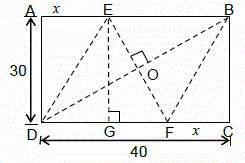The line EF is the fold, which is made such that the corner D meets the diagonally opposite comer B. As DF coincides with FB upon making the fold DF = FB. Similarly, DE = EB. Also, as DF is the part of the length of the rectangle that is being folded so that D coincides with the opposite vertex and BE is the part of the length, that is being folded so that B coincides with D, DF = BE (from symmetry), i.e., quadrilateral DFBE is a rhombus (I) Now assume, FC = x cm

In the right angled triangle △BFC, BF =√BC2 + FC2 = √(30)2 + x2 = DF(since, EDBFis a rhombus and BF = DF)

Hence√302 + x2 = 40 - x

=> 900+x2= 1600 + x2– 80x

=> x = 700 / 80 = 35 / 4

Now, consider G on DC, such that EG ⊥DC. In △EGF, GF = 40 - 2x (as AE = FC = x), EG = 30 and EF is the length of the fold.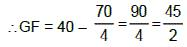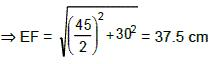Alternative Solution

Consider the conclusion (I), i.e., that EDBF is a rhombus. Let the diagonals of the rhombus meet at O. In a rhombus, the diagonals bisect each other at right angles. Hence △EOB is similar to △DAB (both are right angled, with a common angle at B).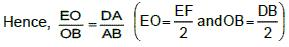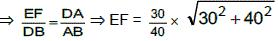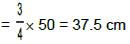QUESTION: 15

DIRECTIONS for questions: Select the correct alternative from the given choices.

M workers started a job. At the end of theith day of the job, where 1 ≤ i ≤ M, the ith worker left the job. The job was completed when the Mth worker left. If the ith worker can do i units of work per day and the job to be done was equal to 204 units of work, find M.

Solution: Part of the job doneonthe first day= (1+ 2 + 3....+M)units

Part of the job doneon the second day =(2+ 3 +…+M)units.

Part of the job done on the mlh day = (m +…+ M) units.

And finally, part of the job done on the Mth day = M units

Job =1+2+ 3+ + M

+ 2 + 3 +….+ M

+ 3……….

+ M

= (12 + 22 + 32…..M2) units

= (M)(M + 1)(2M + 1) / 6 units

=>(M)(M + 1)(2M + 1) / 6 = 204 (given)

=> (M) (M + 1) (2M + 1) = 1224 = (8) (9) (17)

comparing both sides, M = 8

QUESTION: 16

DIRECTIONS for questions: Select the correct alternative from the given choices.

If x and y are positive integers and x2 + y2 = 1800, then the maximum value ofx + y is

Solution: For maximum value of x + y, x and y should be as close as possible i.e., ideally x = y.

If x = y and x2 + y2 = 1800 => x= 30 and y = 30 so maximum value of x + y is 60

QUESTION: 17

DIRECTIONS for questions: Select the correct alternative from the given choices.

There are 2272 students in a school. All the students stand in a row, from left to right, holding a number such that the number with any student (except those at the ends) equals the sum of the numbers with the student on his immediate left and the student on his immediate right. If the numbers with the 1136thand 1137thstudents from the left end are – 57 and 16 respectively, then find the sum of the numbers with all the students.

Solution: Let the number with the ithstudent = Ni

Given Ni = Ni-i + Ni+ i

Hence the number with 1137thstudent

= N1137 = N1138 + N1138

=> 16 = -57 + N1133

=> N1138 = 73

similarly N1138 =-73

and N1139 = 57

and N1134 = -16

and N1140 = -16

∴we see that the series of numbers from N1134 onwards is as follows

-16, -73, -57, 16, 73, 57, -16, -73, -57 and so on, with the set of six values repeating continuously. Note that the sum of these six values themselves is zero.

From the pattern, we can say that the number with the student of the form N = 6k + 1 will be -73.

(∴ N1135 = -73 and 1135 = 6k + 1)

∴we can ignore all students till the highest multiple of six under (or equal to) 2272 i.e, till 2268.

Now only four more students will remain, and they will have the numbers-73,-57,16 and 73.

Hence, the sum of the numbers with them will be -41.

QUESTION: 18

DIRECTIONS for questions: Select the correct alternative from the given choices.

If the areas of the six faces of a cuboid are a1, a2, ……. a6, then the volume of the cuboid is

Solution: Let a1 = a2 = lb,

a3 = a4 = bh,

a5 =a6 =hl.

A1 a2a3 a4a5 a6 =ℓ4 b4 h4 ={ℓbh)4

∴ volume =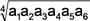QUESTION: 19

DIRECTIONS for questions: Select the correct alternative from the given choices.

If both p and q belong to the set {1, 2, 3, 4}, find the number of equations of the form 2x− px + q = 0, which have real roots.

Solution: As the roots are real, p2 - 4q > 0.

p2> 4q. If q = 1, p can be 2 or 3 or 4.

If q = 2, p can be 3 or 4.

If q = 3, p can be only 4.

If q = 4, p can be only 4.

∴There are 7 possible equations.

QUESTION: 20

DIRECTIONS for questions: Select the correct alternative from the given choices.

In a certain class, there are two sections – A and B – with equal number of students in each section. The average height ofthe students of section A is 155 cms and that of section B is 160 cms. One-fourth of the students from section B move to section A, thereby increasing the average height of section A by 1 cm. What happens to the average height of section B after this movement of students to section A?

Solution:

Let the number of students in each section be 4k.

The sum of the heights of all the students of section A is 4k x 155 = 620 k

The sum of the heights of all the students in section A after 1/4thof the students of section B moved to section A is 5k x 156 = 780k

The sum of the heights of all the students who moved to section A from section B.

780k-620k = 160k

The average height of the students who moved = 160

= 160 cm

As the average height of the students who moved out of section B is the same as the average height of section B, the average height of section B will not change after the movement of students.

QUESTION: 21

DIRECTIONS for questions : Select the correct alternative from the given choices. Given that |x| < 1, find the value of 1 + 4x2+ 9x4 + 16x6 + 25x8 ….∞.

(d)1 + x2 / (1 – x2)3

Solution:

Let the value of 1 + 4x2 + 9X4 + 16x + ... ∞ be denoted by S.

S = 1+4x2 + 9x4+16x6 + … (1)

Sx2 = x2 + 4x4 + 9x6 + … (2)

Subtracting (2) from (1)

S (1 - x2) = 1 + 3x2 + 5x4 + 7x6…(3)

Multiplying (3) by x2, we have

Sx2 (1 - x2) = x2 + 3X4 + 5x6 + … (4)

Subtracting (4) from (3),

S (1 - x2 - x2 + x4) = 1 + 2x2 + 2X4 + 2X8 + ..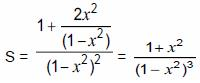QUESTION: 22

DIRECTIONS for questions: Select the correct alternative from the given choices.

The sum (S) of the squares of the first n natural numbers is equal to the sum of the first 2n natural numbers. What is the remainder when S is divided by 2n?

Solution: Given that 1+2 + 3 +….+ 2n = 12+22+ ... + n2=S

⇒ 2n(2n + 1) / 1 = n(n + 1)(2n + 1) / 6 ⇒ n + 1 = 6 ⇒ n = 5

∴S = 5(11) = 55 and the remainder when S is divided by 2n(i.e., 10) is 5.

QUESTION: 23

DIRECTIONS for questions: Select the correct alternative from the given choices.

Water flowing at a speed of 10 m/s, through a cylindrical pipe of length 20 m and diameter 6 cm, can fill a tank of volume V in 2 hours. The volume of the tank that can be completely filled by water flowing at a speed of 20 m/s, through a cylindrical pipe of length 40 m and of radius 4 cm, in 1 hour is

Solution: Volume ∝ (speed) x (Area of cross-section) x (time of flow)

The length of the pipe is irrelevant information for the given question.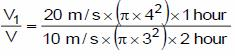= 16 / 9

∴V1 = 16V / 9

QUESTION: 24

DIRECTIONS for questions: Select the correct alternative from the given choices.

If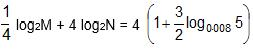, which of the following is true?

Solution: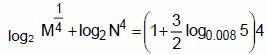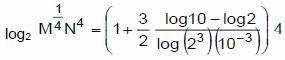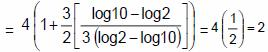∴M 1 / 4 N4 = 4 => MN16 = 256.

QUESTION: 25

DIRECTIONS for questions: Select the correct alternative from the given choices.

The age of a person k years ago was half of what his age would bek years from now. The age of the same personp years from now would be thrice of what his age was p years ago. What is the value of the ratiok : p?

Solution: Let the present age of the person be y years.

The given data can be written as:

(y – k) = 1 / 2 (y + k) --- (1)

(y + P) = 3 (y-p) --- (2)

From (1), y + k / y – k = 2 / 1;

=> y / k = 3 / 1 (by componendo and dividendo) ---(3)

From (2), y + p / y – p = 3 / 1 => y / p = 4 / 2 = 2 ---(4)

Dividing (4) by (3), y / p x k / y = 2 / 3 ; => k : p = 2 : 3